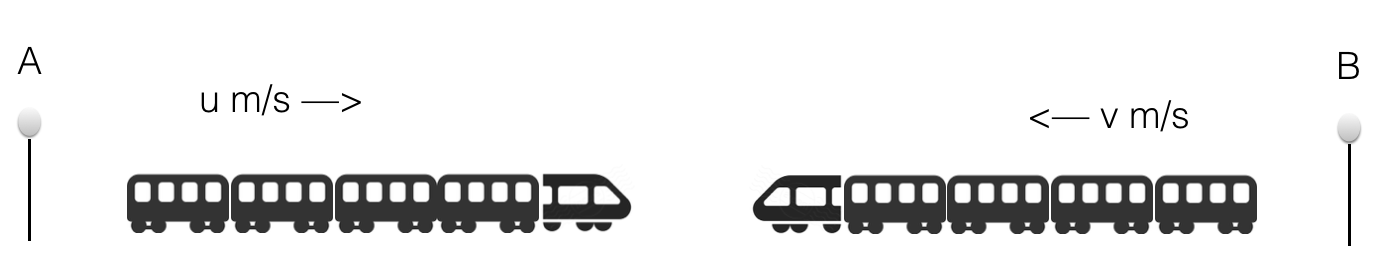# Problem on Trains

Aptitude

Important notes and formula

### Convert km/hr to m/s

 N km/hr = N x 5 m/s 18

### Convert m/s to km/hr

 N m/s = N x 18 km/hr 5

### Crossing a pole

Time taken by a train of length L meters to cross a person standing on a platform, or a signal pole or a lamp post is equal to the time taken by the train to cover L meters.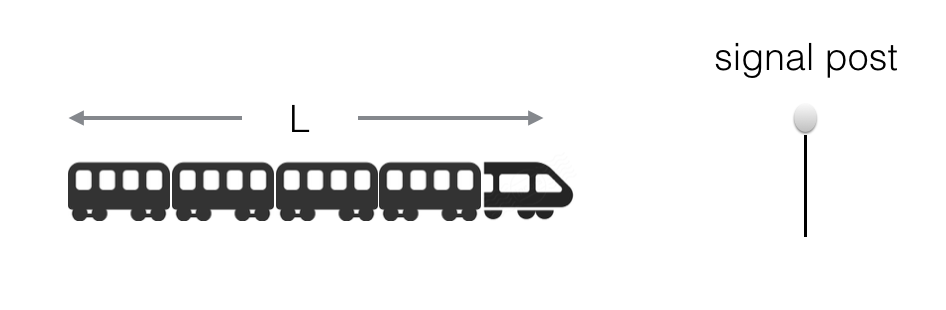### Crossing an object or platform

Time taken by a train of length L meters to cross a platform or object of length X is equal to the time taken by the train to cover (L + X) meters.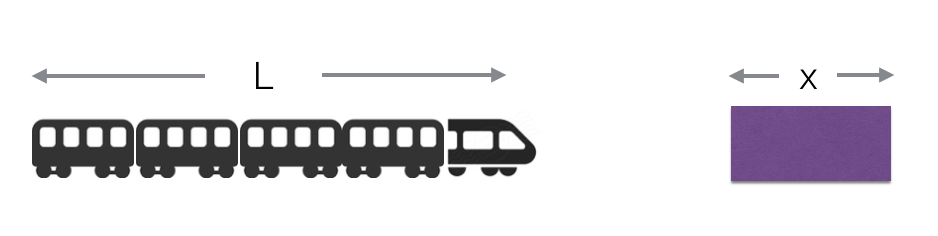### Two trains running in opposite direction

If two trains are running in opposite direction at a speed u m/s and v m/s respectively then their relative speed is (u+v) m/s.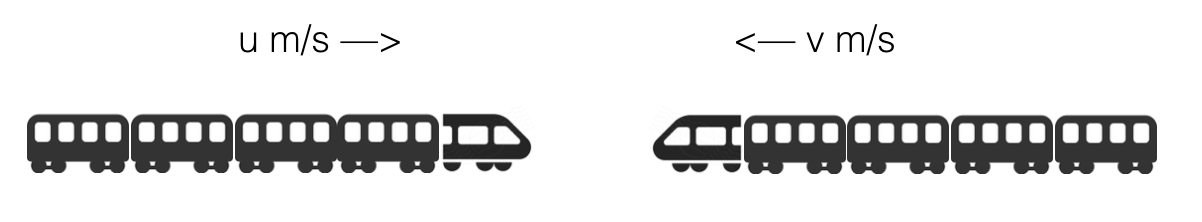### Two trains running in same direction

If two trains are running in same direction at a speed u m/s and v m/s respectively then their relative speed is (u-v) m/s where, u > v.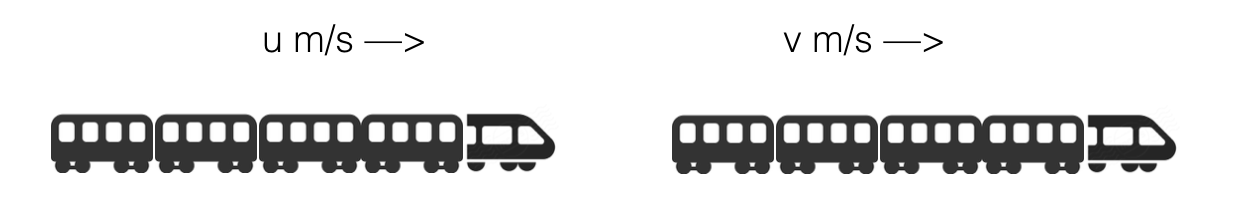### Two trains running in opposite direction (case 2)

If two trains L meters and M meters are running in opposite direction at a speed u m/s and v m/s respectively.
Then time taken by the trains to cross each other is
(L + M) / (u + v) seconds.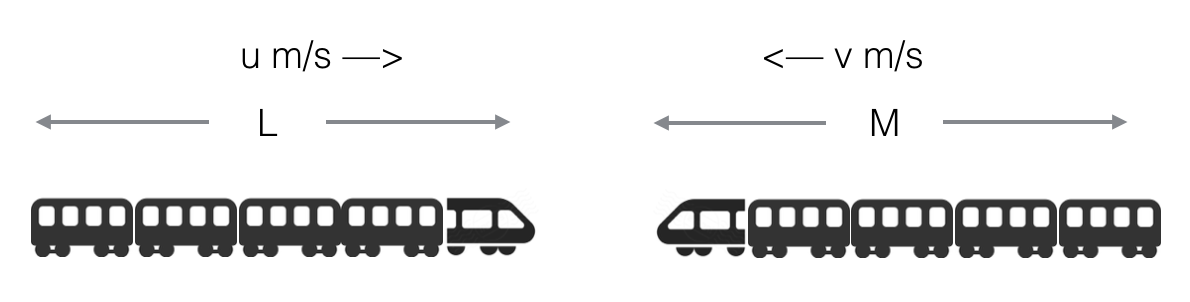### Two trains running in same direction (case 2)

If two trains L meters and M meters are running in same direction at a speed u m/s and v m/s respectively where, u > v.
Then time taken by the faster train to cross the slower train is
(L + M) / (u - v) seconds.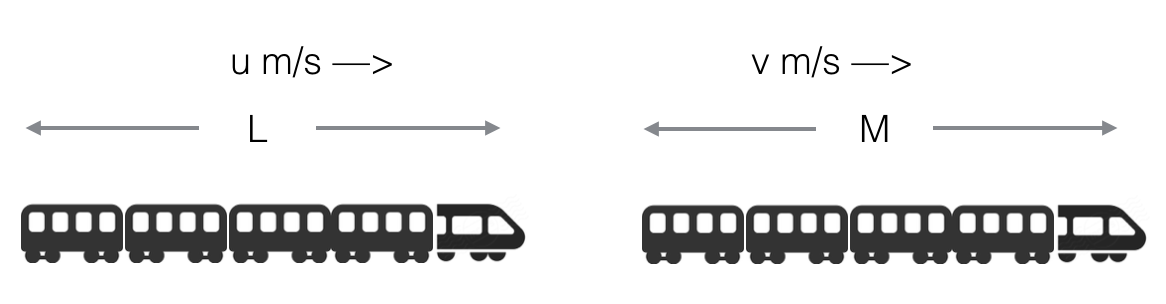### Finding speed

If two trains start from point A and B respectively at the same time and starts approaching each other and after crossing they take x and y seconds respectively to reach point B and A respectively. Then speed of the two trains will be
u : v = √x : √y